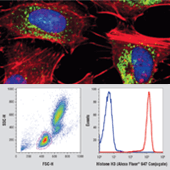Dropping with the temps: Cool deals on CST mAbs | Learn More >>

# Product Results## Direct Conjugated Primary Antibodies and Custom Conjugation Services

Offering primary conjugated antibodies with a variety of conjugation types to meet your needs for Flow Cytometry, IF, Western Blot, and other applications. Our conjugated antibodies are tested and validated on the lot level by our in-house team of scientists for specificity, sensitivity, and reproducibility.

Our custom conjugation group offers price-competitive direct conjugation services of CST antibodies that include extensive technical, assay development and application consultation.

# Product Name Application Reactivity
H
H
H
H
H
H
H
H, M, R, Mk
H, M, R, Mk
H, M, R, Mk
H, M, R, Mk
H, R
H
H
H
H, M, R
H, M, R
H, M, R
H, M, R
H, M, R
H, M, R
H, M, R
H, M, R, Mk, Dm
H, M, R, Mk, Dm
H, M, R, Mk, Dm
H, M, R, Mk, Z, Dg
H, M, R
H, M, R
H, M, R
H, M, R
H, M, R, Mk
H, M, R, Mk
H, M, R, Mk
H, M, R, Mk
H, M, R, Mk
H, M, R
H, M, R, Mk
H, M, Mk
H, M, Mk
H, M, Mk
H, M, R, Mk, B, Dg, Pg
H
H
H, M, R
H, M, R
H, M, R
H
H
H
H, M, R, Mk, Pg
H, M, R, Mk, Pg
H, M, R
H, M, R, Mk
H, M, R
H, M, R
H, M, R
H, M, R, Mk
H, M, R
H, M, R
H, M, R